Implicit intersection has been around in Excel for years, but very few people cared about it. Now that it is no longer the default behavior in Excel 365, many questions arise. This tutorial aims to explain the meaning of the @ sign in Excel formulas, which is called an implicit intersection operator.

You have the feeling you know everything about your workbooks, and then a @ character appears from nowhere in the beginning of your formulas. What does this @ mean? And how does it work precisely? In a nutshell, it is an implicit intersection operator that disables the new default array behavior of a formula and tells Excel to return a single value. For more detail, please continue reading.

## Excel implicit intersection

Implicit intersection in Excel means reducing multiple values to a single value. Normally, it occurs when an array or range is supplied to a formula that is supposed to output just one value in a single cell.

### Implicit intersection in Excel 2019 - 2000

In traditional Excel, implicit intersection is the default behavior. It is silently performed in the background for all formulas. The implicit intersection logic is as follows:

• If the formula produces a single value, return that value (in fact, implicit intersection does nothing in this case).
• In case of a range, use a value from the cell on the same row or column as the formula.
• In case of an array, use the top-left value.

For example, when multiplying two columns of numbers, Excel picks just one number from each column in the same row where the formula is and outputs the result only in one cell (D2 in our case):

`=B2:B5*C2:C5`

To multiply the numbers in other cells, you need to copy the formula down.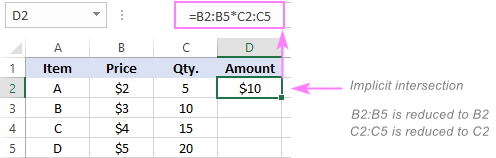To disable implicit intersection, you must enter an array formula with Ctrl + Shift + Enter (which is why traditional array formulas are sometimes called CSE formulas). This clearly tells Excel to process multiple values input as ranges or arrays.

In our case, select cells D2:D5, type the above formula and confirm it by pressing the Ctrl + Shift + Enter keys together. Once you do this, the formula gets surrounded in {curly braces}, indicating it's an array formula. As the result, the numbers in each row get multiplied at once: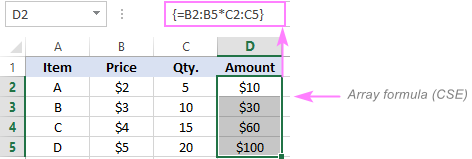### Implicit intersection in Excel 365

The introduction of dynamic arrays has changed the default behavior of all formulas in Excel 365. Now, any formula that can potentially produce multiple results, automatically spills them onto the sheet. That makes implicit intersection unnecessary, and it is no longer triggered by default. For this reason, Excel 365 is sometimes called dynamic array Excel or DA Excel.

Here, the entire ranges are multiplied with a regular formula that is only entered in the topmost cell (D2):

`=B2:B5*C2:C5`

The result is a spill range consisting of 4 cells: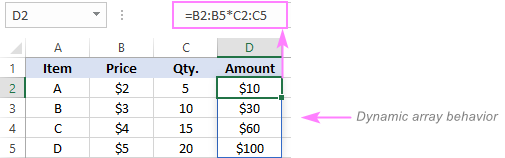If you want a formula to return just one value, you need to explicitly enable implicit interception. For this, they introduced a special operator, and in the next section you will find full details about it.

The implicit intersection operator was introduced in Excel 365 to prevent the default dynamic array behavior. If you wish a formula to return just one value, put @ before the function's name (or before a certain range or array inside the formula), and it will behave like a regular non-array formula in pre-dynamic versions.

For example:

`=@B2:B5*@C2:C5`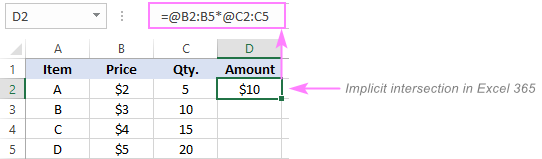Note. The implicit intersection operator is only supported in Microsoft 365 subscriptions. If you try to add the @ sign in older versions, it will be silently removed upon completing the formula.

### Why is @ added to old formulas?

In Excel 365, you may notice the @ character added to some of your formulas when opening a workbook created in an older version. For the most part, it is done to force a formula to behave the same way as it did in the original version in which it was created. In other words, if a formula returned a single value in an older version but would return multiple results in Excel 365, it will be automatically prefixed with @ to deactivate the array behavior.

Typically, the intersection operator is inserted before the function that could return multi-cell arrays or ranges such as OFFSET, INDEX, or user-defined functions.

For example, the following formula authored in pre-dynamic Excel:

`=INDEX(B2:C5,,F1)`

will take the following form in dynamic array Excel:

`=@INDEX(B2:C5,,F1)`

The reason is that without the @ operator, the formula will return all the values from C2:C5 because the row_num argument of the INDEX function is omitted. To ensure the consistent behavior in all versions, the previously unnoticeable implicit intersection becomes evident. Please compare the results: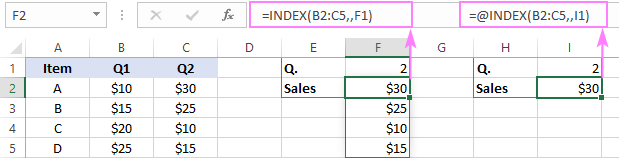In case a function with a potential multi-cell output is nested in another one that can handle arrays and output a single result (such as SUM, COUNT, AVERAGE, etc.), then there is no reason to trigger implicit intersection, and the formula is transferred to dynamic Excel as-is without adding the @ sign. For example:

`=AVERAGE(INDEX(B2:C5,,F1))`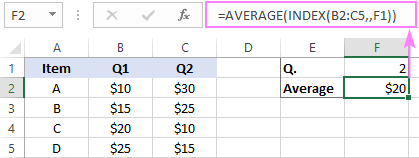### Can I remove the @ symbol from my formulas?

Sure, you can. Excel performs the formula conversion just once. If the @ operator breaks or negatively changes the behavior of your formulas, you can delete @ manually, and it won't appear again after saving the workbook.

What are the consequences of deleting the implicit intersection operator? Depending on what the part of the formula following the @ sign returns, there are three possible outcomes:

• If a single value is returned, there will be no change.
• If an array is returned, it will spill into the adjacent cells.
• If an array is returned but there are not enough empty cells to display all the values, a #SPILL error will occur.

### Why use the @ operator in Excel 365?

As already mentioned, Excel for Microsoft 365 treats all formulas as array formulas by default, without you having to press Ctrl + Shift + Enter like you used to do in the previous versions. If you want to disable the array behavior, then insert the implicit intersection operator.

#### Example 1. Get rid of #SPILL! error

A very common scenario is preventing or fixing #SPILL errors. If you find it convenient to refer to entire columns (which is generally not a very good idea because it slows down Excel), but nevertheless such a formula will work fine in pre-dynamic versions:

`=VLOOKUP(A:A, D:E, 2, FALSE)`

In dynamic Excel, it will result in a #SPILL error because there isn't enough space to display nearly 1.05 million results.

Adding @ before the lookup_value argument resolves the problem:

`=VLOOKUP(@A:A, D:E, 2, FALSE)`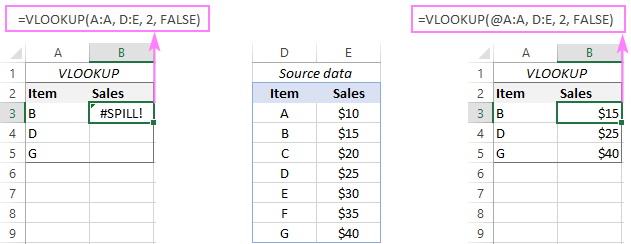When Excel foresees that the formula may spill beyond the edges of the worksheet, it will suggest a correction, and you would be wise to accept it: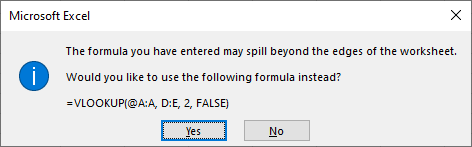#### Example 2. Get the formula to work properly in older Excel

If you are using a Microsoft 365 subscription and share your files with someone who sticks with an older version, it's important to ensure the consistent formula behavior for everyone.

Suppose you have written this dynamic array formula in Excel 365:

`=B2:B5*C2:C5`

In older versions, it will be converted to a legacy CSE array formula:

`{=B2:B5*C2:C5}`

and return a range of values in both cases: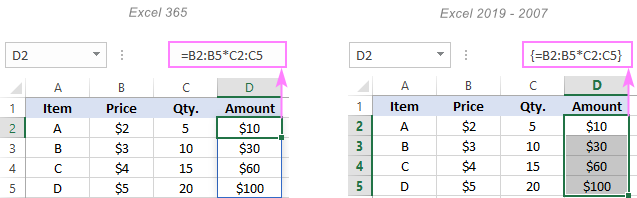If you wish to output just one result, add @ before each expression, and then copy the formula to as many cells as needed (to keep the ranges unchanged, remember to lock them with absolute cells references):

`=@\$B\$2:\$B\$5*@\$C\$2:\$C\$5`

In older Excel, the @ symbol will be automatically removed, and the formula will take a normal look:

`=\$B\$2:\$B\$5*\$C\$2:\$C\$5`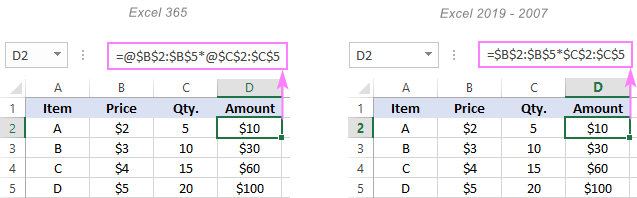What you should not do is mix implicit intersection and array calculations within one formula! For example, if you try to enter something like this in Excel 365:

`=@B2:B5*C2:C5`

You will be notified that such a formula is not supported in older versions: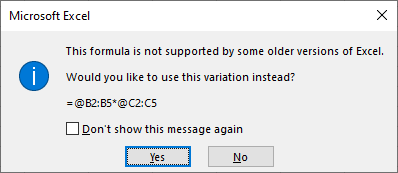If you reject the proposed variation, the mixed formula will be committed and will deliver some results (though, it may not be the results you expected). But when you open that formula in pre-dynamic Excel, the _xlfn.SINGLE function will appear instead of the implicit intersection operator:

`=_xlfn.SINGLE(B2:B5)*C2:C5`

When this formula is evaluated by older Excel, a #NAME! error will be returned.

## Implicit intersection in Excel tables

Generally speaking, the implicit intersection behavior in tables is consistent with your Excel version.

In Excel 2019 and earlier, you can refer to a whole column, and the formula will resolve to a single cell in the current row.

For example, this formula successfully multiplies the numbers in the Price and Qty. columns:

`=[Price]*[Qty.]`

Even though it refers to the entire columns, pre-dynamic Excel still operates on single values at a row level.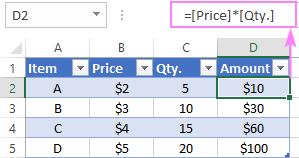In Excel 365, this approach won't work because implicit intersection is off by default. Because the formula returns multiple values and Excel is unable to fit them all into a table, it will suggest prefixing the column names with the @ symbol: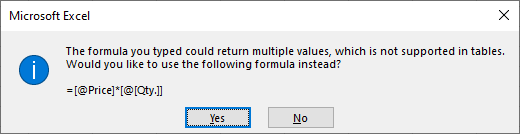If you have experience with table references, this syntax should be familiar to you - the @ symbol indicates that the formula will process the values from columns Price and Qty. on the same row.

`=[@Price]*[@[Qty.]]`

And it will work nicely in all versions: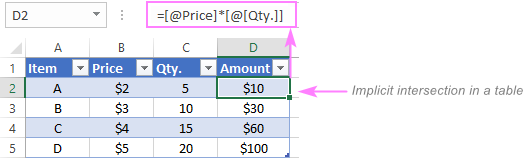Tip. If you intend to use a dynamic array function to calculate a table's data, be sure to place your formula outside the table, because inside tables dynamic arrays are not supported.

That's how implicit intersection works in Excel. I thank you for reading and hope to see you on our blog next week!

## You may also be interested in:

1. office 365/excel - situation with the following formula:
=MEDIAN(IF(C5:C13=C1,B5:B13))
excel adds implicit intersection operator "@" and cell is populated with #VALUES
unable to remove "@" - "@" reappears after it is removed:
settings issue? settings/build issue?

2. Your blogs are all so excellent and useful. Thank you very much Svetlana

3. Good article!

I guess this note in your text may need an update: "Note. The implicit intersection operator is only supported in Microsoft 365 subscriptions. If you try to add the @ sign in older versions, it will be silently removed upon completing the formula." Can you tell me in what non-subscription stand-alone-version of Excel dynamic arrays were introduced? Was it 2019 or 2021?

4. Hi. I want to disable the Spill function so that my formula only returns a value in a specific cell. I've tried using @ (e.g. =@D24:D26) but it's returning a #VALUE error. Please could you help? Thanks.

• Hi!
This formula works on row 24.

5. useless implicit operator @, if this show my index match is useless unless i delete this useless implicit operator

6. Hello,
Thanks for your article - very usefull
I have a problem - i wrote a regular formula in excel 365. when my parthner open the file in office 2019 it's gets curly brake ( {} ) and become array formula that returned an error.
how can slove this problem?
thanks
Guy

• Hello Guy,

In Excel 365, any formula that can potentially return multiple values or perform multiple calculations is a dynamic array formula by default.

To ensure the consistent formula behavior in all versions, Excel automatically converts dynamic array formulas into traditional CSE array formulas (enclosed in curly braces) when a workbook created in Excel 365 is opened in non-dynamic Excel (2019 and older).

Without seeing your formula, it's not possible to determine the root cause of the error. If you can post the formula here, we will try to figure it out.

7. Hi Svetlana , i like your article, I requested u please write an article on Lambda Function.

• Thank you for your feedback! Lambda is already on my to-do list :)

• I am happy to report that our LAMBDA tutorial is published. Hopefully, you'll find it helpful.